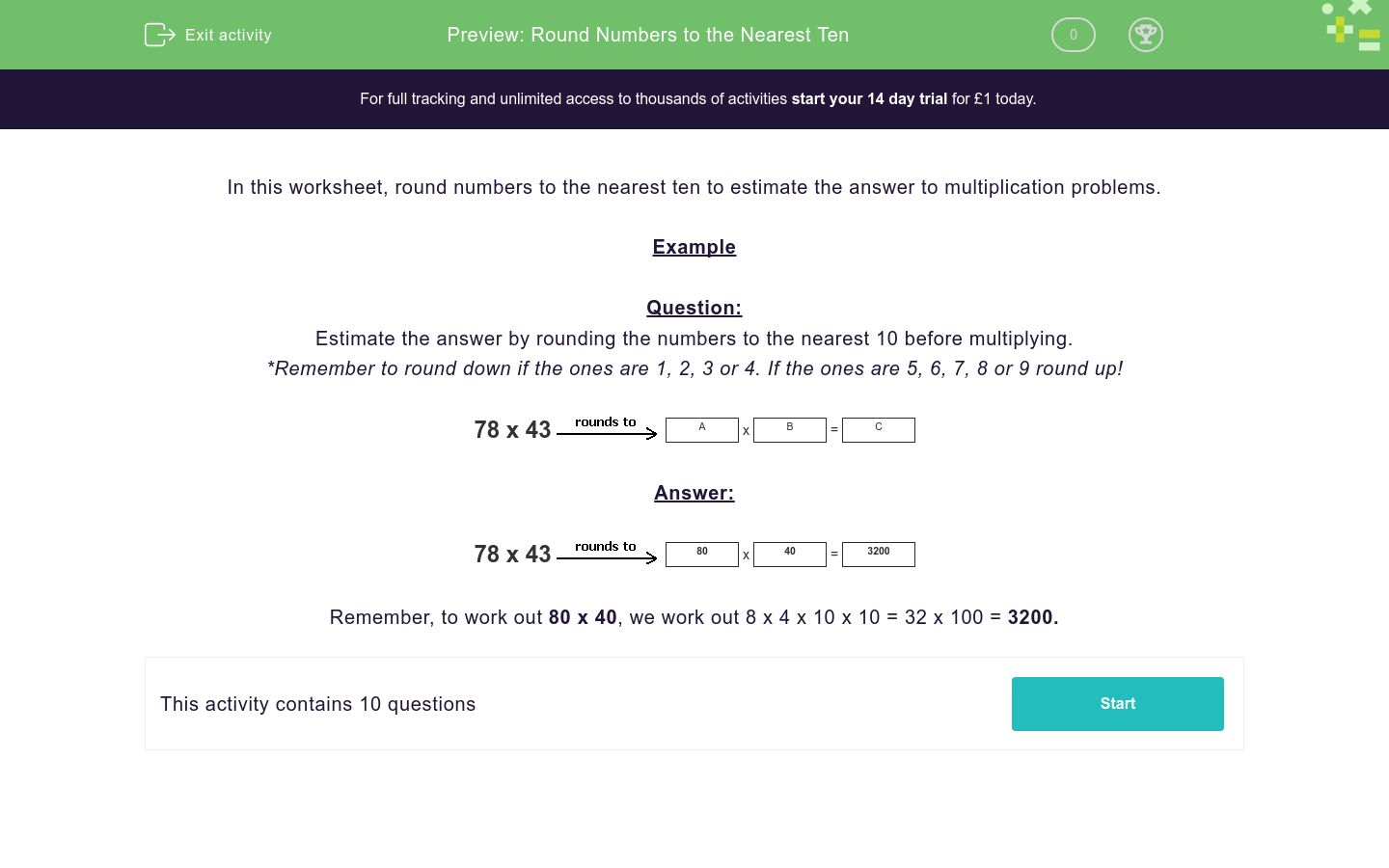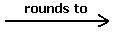# Round Numbers to the Nearest Ten

In this worksheet, students round numbers to the nearest 10 before multiplying to find an estimated answer.Key stage:  KS 2

Curriculum topic:   Number: Addition and Subtraction

Curriculum subtopic:   Use Rounding for Answers/Accuracy

Difficulty level:### QUESTION 1 of 10

In this worksheet, round numbers to the nearest ten to estimate the answer to multiplication problems.

Example

Question:

Estimate the answer by rounding the numbers to the nearest 10 before multiplying.

*Remember to round down if the ones are 1, 2, 3 or 4. If the ones are 5, 6, 7, 8 or 9 round up!

78 x 43A
x
 B
=
 C

78 x 4380
x
 40
=
 3200

Remember, to work out 80 x 40, we work out 8 x 4 x 10 x 10 = 32 x 100 = 3200.

Estimate the answer by rounding the numbers to the nearest 10 before multiplying to find C.

67 x 32A
x
 B
=
 C

## Column B

A
30
B
70
C
2100

Estimate the answer by rounding the numbers to the nearest 10 before multiplying to find C.

59 x 32A
x
 B
=
 C

## Column B

A
60
B
1800
C
30

Estimate the answer by rounding the numbers to the nearest 10 before multiplying to find C.

50 x 28A
x
 B
=
 C

## Column B

A
1500
B
30
C
50

Estimate the answer by rounding the numbers to the nearest 10 before multiplying to find C.

37 x 20A
x
 B
=
 C

## Column B

A
800
B
40
C
20

Estimate the answer by rounding the numbers to the nearest 10 before multiplying to find C.

73 x 30A
x
 B
=
 C

## Column B

A
30
B
2100
C
70

Estimate the answer by rounding the numbers to the nearest 10 before multiplying to find C.

31 x 28A
x
 B
=
 C

## Column B

A
30
B
900
C
30

Estimate the answer by rounding the numbers to the nearest 10 before multiplying to find C.

43 x 19A
x
 B
=
 C

## Column B

A
40
B
800
C
20

Estimate the answer by rounding the numbers to the nearest 10 before multiplying to find C.

36 x 29A
x
 B
=
 C

## Column B

A
30
B
40
C
1200

Estimate the answer by rounding the numbers to the nearest 10 before multiplying to find C.

97 x 83A
x
 B
=
 C

## Column B

A
8000
B
100
C
80

Estimate the answer by rounding the numbers to the nearest 10 before multiplying to find C.

27 x 12A
x
 B
=
 C

## Column B

A
30
B
10
C
300
• Question 1

Estimate the answer by rounding the numbers to the nearest 10 before multiplying to find C.

67 x 32A
x
 B
=
 C

## Column B

A
70
B
30
C
2100
EDDIE SAYS
Round 67 up to 70. Round 32 down to 30.
• Question 2

Estimate the answer by rounding the numbers to the nearest 10 before multiplying to find C.

59 x 32A
x
 B
=
 C

## Column B

A
60
B
30
C
1800
EDDIE SAYS
Round 59 up to 60. Round 32 down to 30.
• Question 3

Estimate the answer by rounding the numbers to the nearest 10 before multiplying to find C.

50 x 28A
x
 B
=
 C

## Column B

A
50
B
30
C
1500
EDDIE SAYS
50 stays the same. Round 28 up to 30.
• Question 4

Estimate the answer by rounding the numbers to the nearest 10 before multiplying to find C.

37 x 20A
x
 B
=
 C

## Column B

A
40
B
20
C
800
EDDIE SAYS
Round 37 up to 40. 20 stays the same.
• Question 5

Estimate the answer by rounding the numbers to the nearest 10 before multiplying to find C.

73 x 30A
x
 B
=
 C

## Column B

A
70
B
30
C
2100
EDDIE SAYS
Round 73 rounds down to 70. 30 stays the same.
• Question 6

Estimate the answer by rounding the numbers to the nearest 10 before multiplying to find C.

31 x 28A
x
 B
=
 C

## Column B

A
30
B
30
C
900
EDDIE SAYS
Round 28 up to 30. Round 31 down to 30.
• Question 7

Estimate the answer by rounding the numbers to the nearest 10 before multiplying to find C.

43 x 19A
x
 B
=
 C

## Column B

A
40
B
20
C
800
EDDIE SAYS
Round 19 up to 20. Round 43 down to 40.
• Question 8

Estimate the answer by rounding the numbers to the nearest 10 before multiplying to find C.

36 x 29A
x
 B
=
 C

## Column B

A
40
B
30
C
1200
EDDIE SAYS
Round 36 up to 40. Round 29 up to 30.
• Question 9

Estimate the answer by rounding the numbers to the nearest 10 before multiplying to find C.

97 x 83A
x
 B
=
 C

## Column B

A
100
B
80
C
8000
EDDIE SAYS
Round 97 up to 100. Round 83 down to 80.
• Question 10

Estimate the answer by rounding the numbers to the nearest 10 before multiplying to find C.

27 x 12A
x
 B
=
 C

## Column B

A
30
B
10
C
300
EDDIE SAYS
Round 27 up to 30. Round 12 down to 10.
---- OR ----

Sign up for a £1 trial so you can track and measure your child's progress on this activity.

### What is EdPlace?

We're your National Curriculum aligned online education content provider helping each child succeed in English, maths and science from year 1 to GCSE. With an EdPlace account you’ll be able to track and measure progress, helping each child achieve their best. We build confidence and attainment by personalising each child’s learning at a level that suits them.

Get started